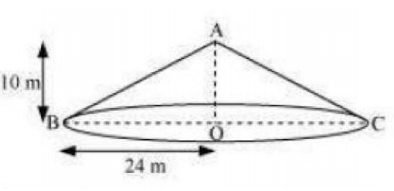# NCERT Solutions Class 9 Mathematics Solutions for Surface Areas and Volumes - Exercise 13.3 in Chapter 13 - Surface Areas and Volumes

A conical tent is 10 m high and the radius of its base is 24 m. Find

(i) slant height of the tent.

(ii)cost of the canvas required to make the tent, if the cost of 1 m2 canvas is Rs 70.

(Assume π=22/7)Let ABC be a conical tent

Height of conical tent, h = 10 m

The radius of a conical tent, r = 24 m

Let the slant height of the tent be l.

(i)In the right triangle, ABO,

\begin{aligned} &\mathrm{AB}^{2}=\mathrm{AO}^{2}+\mathrm{BO}^{2} \text { (using Pythagoras theorem) }\\ &l^{2}=h^{2}+r^{2}\\ &=(10)^{2}+(24)^{2}\\ &=676 \end{aligned}

l = 26

Therefore, the slant height of the tent is 26 m.

(ii) CSA of tent = πrl

\begin{aligned} &=(22 / 7 \times 24 \times 26) \mathrm{m}^{2}\\ &\text { Cost of } 1 \mathrm{m}^{2} \text { canvas }=\mathrm{Rs} 70\\ &\text { cost of }(13728 / 7) \mathrm{m}^{2} \text { canvas is equal to } \mathrm{Rs}(13728 / 7) \times 70=\mathrm{Rs} 137280 \end{aligned}

Therefore, the cost of the canvas required to make such a tent is Rs 137280.

Related Questions

Lido

Courses

Teachers

Book a Demo with us

Syllabus

Maths
CBSE
Maths
ICSE
Science
CBSE

Science
ICSE
English
CBSE
English
ICSE
Coding

Terms & Policies

Selina Question Bank

Maths
Physics
Biology

Allied Question Bank

Chemistry
Connect with us on social media!